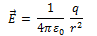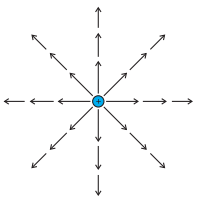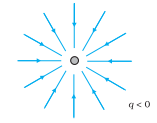# Electric Field Lines – Definition, Properties, Attraction & Repulsion

0
144

## What is Electric Field Line?

Electric field lines are an excellent way of visualizing electric fields. They were first introduced by Michael Faraday himself.

A field line is drawn tangential to the net at a point. Thus at any point, the tangent to the electric field line matches the direction of the electric field at that point. Secondly, the relative density of field lines around a point corresponds to the relative strength (magnitude) of the electric field at that point. In other words, if you see more electric field lines in the vicinity of point A as compared to point B, then the electric field is stronger at point A.

## Properties of Electric Field Lines

• The field lines never intersect each other.
• The field lines are perpendicular to the surface of the charge.
• The magnitude of charge and the number of field lines, both are proportional to each other.
• The start point of the field lines is at the positive charge and end at the negative charge.
• For the field lines to either start or end at infinity, a single charge must be used.

For a single positive point charge q, electric field is• Since electric field varies as the inverse of the square of the distance that points from the charge the vector gets shorter as you go away from the origin and they always points radially outwards.
• Connecting up these vectors to form a line is a nice way to represent this field.
• The magnitude of the field is indicated by the density of the field lines.
• Magnitude is strong near the center where the field lines are close together, and weak farther out, where they are relatively apart.
• So, electric field line is an imaginary line drawn in such a way that its direction at any point is same as the direction of the field at that point.
• An electric field line is, in general, a curve drawn in such a way that the tangent to it at each point in the direction of the net field at that point.
• Field lines of a single position charge point radially outwards while that of a negative charge is radially inwards as shown below in the figure.• Field lines around the system of two positive charges give a different picture and describe the mutual repulsion between them.• Field lines around a system of a positive and negative charge clearly show the mutual attraction between them as shown below in the figure.• Some important general properties of field lines are
• Field lines start from positive charge and end on a negative charge.
• Field lines never cross each other if they do so then at the point of intersection there will be two directions of the electric field.
• Electric field lines do not pass through a conductor, this shows that electric field inside a conductor is always zero.
• Electric field lines are continuous curves in a charge-free region.